# Home Work Set # 10, Physics 217, Due: December 5, 2001

### Problem 1

Find the force of attraction between two magnetic dipoles,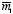and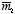, oriented as shown in Figure 1, a distance d apart, using
(a) equation (6.2) of Griffiths.
(b) equation (6.3) of Griffiths.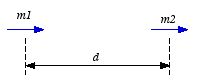Figure 1. Problem 1.

### Problem 2

A uniform current density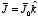fills a slab straddling the yz plane, from x = -a to x = +a. A magnetic dipole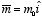is situated at the origin.
a) Find the force on the dipole using equation (6.3) of Griffiths.
b) Do the same for a dipole pointing in the y-direction: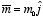.

### Problem 3

A long circular cylinder of radius R carries a magnetization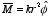, where k is a constant, r is the distance from the axis, and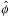is the azimuthal unit vector. Find the magnetic field due tofor points inside and outside the cylinder.

### Problem 4

A short circular cylinder of radius R and length L carries a "frozen-in" uniform magnetization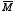parallel to its axis. Find the bound current, and sketch the magnetic field of the cylinder. (Make two sketches: one for L >> R, and one for L << R.)

### Problem 5

Of the following materials, which would you expect to be paramagnetic and which diamagnetic? Aluminum, copper, copper chloride (CuCl2), carbon, lead, nitrogen (N2), salt (NaCl), sodium, water.